# Flip-Flop Circuits

## Digital Circuits

• #### Question 1

 Don’t just sit there! Build something!!

Learning to analyze digital circuits requires much study and practice. Typically, students practice by working through lots of sample problems and checking their answers against those provided by the textbook or the instructor. While this is good, there is a much better way.

You will learn much more by actually building and analyzing real circuits, letting your test equipment provide the “answers” instead of a book or another person. For successful circuit-building exercises, follow these steps:

1. Draw the schematic diagram for the digital circuit to be analyzed.
2. Carefully build this circuit on a breadboard or other convenient medium.
3. Check the accuracy of the circuit’s construction, following each wire to each connection point, and verifying these elements one-by-one on the diagram.
4. Analyze the circuit, determining all output logic states for given input conditions.
5. Carefully measure those logic states, to verify the accuracy of your analysis.
6. If there are any errors, carefully check your circuit’s construction against the diagram, then carefully re-analyze the circuit and re-measure.

Always be sure that the power supply voltage levels are within specification for the logic circuits you plan to use. If TTL, the power supply must be a 5-volt regulated supply, adjusted to a value as close to 5.0 volts DC as possible.

One way you can save time and reduce the possibility of error is to begin with a very simple circuit and incrementally add components to increase its complexity after each analysis, rather than building a whole new circuit for each practice problem. Another time-saving technique is to re-use the same components in a variety of different circuit configurations. This way, you won’t have to measure any component’s value more than once.

• #### Question 2

A common topology for DC-AC power converter circuits uses a pair of transistors to switch DC current through the center-tapped winding of a step-up transformer, like this: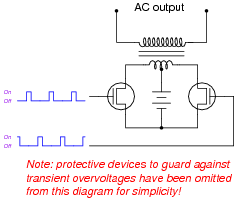In order for this form of circuit to function properly, the transistor “firing” signals must be precisely synchronized to ensure the two are never turned on simultaneously. The following schematic diagram shows a circuit to generate the necessary signals:Explain how this circuit works, and identify the locations of the frequency control and pulse duty-cycle control potentiometers.

• #### Question 3

A common type of rotary encoder is one built to produce a quadrature output: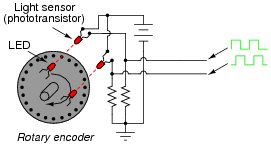The two LED/phototransistor pairs are arranged in such a way that their pulse outputs are always 90o out of phase with each other. Quadrature output encoders are useful because they allow us to determine direction of motion as well as incremental position.

Building a quadrature direction detector circuit is easy, if you use a D-type flip-flop:Analyze this circuit, and explain how it works.

• #### Question 4

Determine the final output states over time for the following circuit, built from D-type gated latches: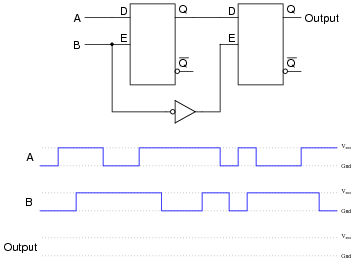At what specific times in the pulse diagram does the final output assume the input’s state? How does this behavior differ from the normal response of a D-type latch?

• #### Question 5

Usually, propagation delay is considered an undesirable characteristic of logic gates, which we simply have to live with. Other times, it is a useful, even necessary, trait. Take for example this circuit: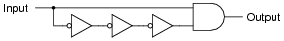If the gates constituting this circuit had zero propagation delay, it would perform no useful function at all. To verify this sad fact, analyze its steady-state response to a “low” input signal, then to a “high” input signal. What state is the AND gate’s output always in?

Now, consider propagation delay in your analysis by completing a timing diagram for each gate’s output, as the input signal transitions from low to high, then from high to low: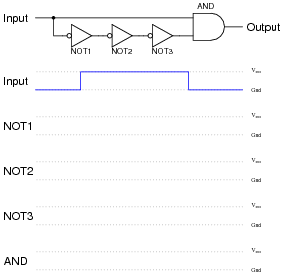What do you notice about the state of the AND gate’s output now?

• #### Question 6

Explain how you would use an oscilloscope to measure the propagation delay of a semiconductor logic gate. Draw a schematic diagram, if necessary. Are the propagation delay times typically equal for a digital gate transitioning from “low” to “high”, versus from “high” to “low”? Consult datasheets to substantiate your answer.

Also, comment on whether or not electromechanical relays have an equivalent parameter to propagation delay. If so, how do you suppose the magnitude of a relay’s delay compares to that of a semiconductor gate, and why?

• #### Question 7

Determine the Q and [Q] output states of this D-type gated latch, given the following input conditions: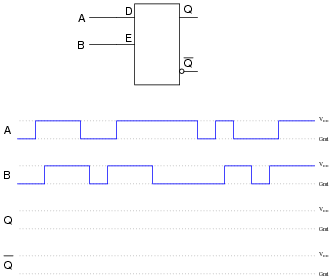Now, suppose we add a propagation-delay-based one-shot circuit to the Enable line of this D-type gated latch. Re-analyze the output of the circuit, given the same input conditions: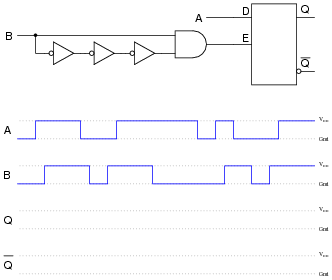Comment on the differences between these two circuits’ responses, especially with reference to the enabling input signal (B).

• #### Question 8

Shown here are two digital components: a D-type latch and a D-type flip-flop:Other than the silly name, what distinguishes a “flip-flop” from a latch? How do the two circuits differ in function?

• #### Question 9

Explain how the addition of a propagation-delay-based one-shot circuit to the enable input of an S-R latch changes its behavior:• #### Question 10

Plain S-R latch circuits are “set” by activating the S input and de-activating the R input. Conversely, they are “reset” by activating the R input and de-activating the S input. Gated latches and flip-flops, however, are a little more complex:Describe what input conditions have to be present to force each of these multivibrator circuits to set and to reset.

For the S-R gated latch:

Set by . . .
Reset by . . .

For the S-R flip-flop:

Set by . . .
Reset by . . .
• #### Question 11

Determine the output states for this S-R flip-flop, given the pulse inputs shown: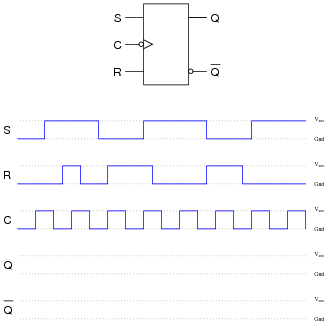• #### Question 12

An extremely popular variation on the theme of an S-R flip-flop is the so-called J-K flip-flop circuit shown here:Note that an S-R flip-flop becomes a J-K flip-flop by adding another layer of feedback from the outputs back to the enabling NAND gates (which are now three-input, instead of two-input). What does this added feedback accomplish? Express your answer in the form of a truth table.

One way to consider the feedback lines going back to the first NAND gates is to regard them as extra enable lines, with the Q and [Q] outputs selectively enabling just one of those NAND gates at a time.

• #### Question 13

Determine what input conditions are necessary to set, reset, and toggle these two J-K flip-flops:For the J-K flip-flop with active-high inputs:

Set by . . .
Reset by . . .
Toggle by . . .

For the J-K flip-flop with active-low inputs:

Set by . . .
Reset by . . .
Toggle by . . .
• #### Question 14

Determine the output states for this J-K flip-flop, given the pulse inputs shown: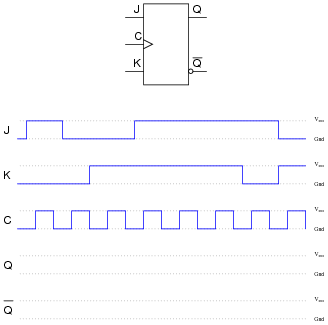• #### Question 15

Determine the output states for this D flip-flop, given the pulse inputs shown: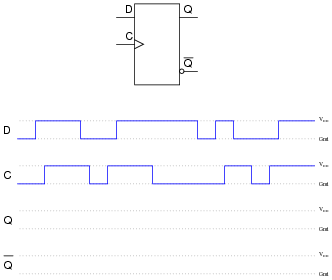• #### Question 16

Determine the output states for this J-K flip-flop, given the pulse inputs shown: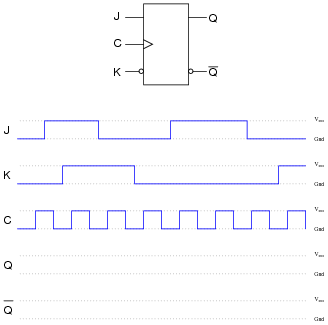• #### Question 17

Flip-flops often come equipped with asynchronous input lines as well as synchronous input lines. This J-K flip-flop, for example, has both “preset” and “clear” asynchronous inputs:Describe the functions of these inputs. Why would we ever want to use them in a circuit? Explain what the “synchronous” inputs are, and why they are designated by that term.

Also, note that both of the asynchronous inputs are active-low. As a rule, asynchronous inputs are almost always active-low rather than active-high, even if all the other inputs on the flip-flop are active-high. Why do you suppose this is?

• #### Question 18

A scientist is using a microprocessor system to monitor the boolean (“high” or “low”) status of a particle sensor in her high-speed nuclear experiment. The problem is, the nuclear events detected by the sensor come and go much faster than the microprocessor is able to sample them. Simply put, the pulses output by the sensor are too brief to be “caught” by the microprocessor every time: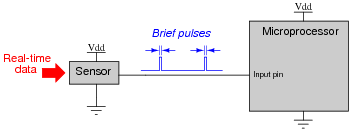She asks several technicians to try and fix the problem. One tries altering the microprocessor’s program to achieve a faster sampling rater, to no avail. Another recalibrates the particle sensor to react slower, but this only results in missed data (because the real world data does not slow down accordingly!). No solution tried so far works, because the fundamental problem is that the microprocessor is just too slow to “catch” the extremely short pulse events coming from the particle sensor. What is required is some kind of external circuit to “read” the sensor’s state at the leading edge of a sample pulse, and then hold that digital state long enough for the microprocessor to reliably register it.

Finally, another electronics technician comes along and proposes this solution, but then goes on vacation, leaving you to implement it: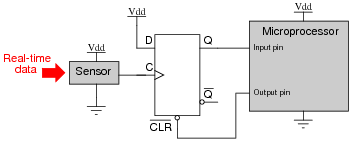Explain how this D-type flip-flop works to solve the problem, and what action the microprocessor has to take on the output pin to make the flip-flop function as a detector for multiple pulses.

• #### Question 19

Identify at least one component fault that would cause the flip-flop to indicate “clockwise” all the time, regardless of encoder motion:For each of your proposed faults, explain why it will cause the described problem.

• #### Question 20

Suppose a student wants to build a sound-controlled lamp control circuit, whereby a single clap or other loud burst of noise turns the lamp on, and another single clap turns it off. The sound-detection and lamp-drive circuitry is shown here:Add a J-K flip-flop to this schematic diagram to implement the toggling function.

• #### Question 21

If the clock frequency driving this flip-flop is 240 Hz, what is the frequency of the flip-flop’s output signals (either Q or [Q])?• #### Question 22

The flip-flop circuit shown here is classified as synchronous because both flip-flops receive clock pulses at the exact same time:Define the following parameters:

Set-up time
Hold time
Propagation delay time
Minimum clock pulse duration

Then, explain how each of these parameters is relevant in the circuit shown.

• #### Question 23

Locate a manufacturer’s datasheet for a flip-flop IC, and research the following parameters:

Flip-flop type (S-R, D, J-K)
Part number
ANSI/IEEE standard symbol
How many asynchronous inputs
Minimum setup and hold times (shown in timing diagrams)
• #### Question 24

Although the toggle function of the J-K flip-flop is one of its most popular uses, this is not the only type of flip-flop capable of performing a toggle function. Behold the surprisingly versatile D-type flip-flop configured to do the same thing:Explain how this circuit performs the “toggle” function more commonly associated with J-K flip-flops.

• #### Question 25

A student has an idea to make a J-K flip-flop toggle: why not just connect the J, K, and Clock inputs together and drive them all with the same square-wave pulse? If the inputs are active-high and the clock is positive edge-triggered, the J and K inputs should both go “high” at the same moment the clock signal transitions from low to high, thus establishing the necessary conditions for a toggle (J=1, K=1, clock transition):Unfortunately, the J-K flip-flop refuses to toggle when this circuit is built. No matter how many clock pulses it receives, the Q and [Q] outputs remain in their original states - the flip-flop remains “latched.” Explain the practical reason why the student’s flip-flop circuit idea will not work.

• #### Question 26

Predict how the operation of this sound-activated lamp circuit will be affected as a result of the following faults. Consider each fault independently (i.e. one at a time, no multiple faults):Resistor R1 fails open:
Resistor R3 fails open:
Diode D1 fails open:
Transistor Q2 fails shorted between collector and emitter:
Solder bridge past resistor R5:

For each of these conditions, explain why the resulting effects will occur.